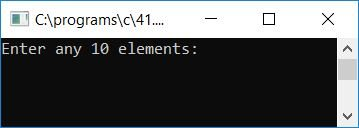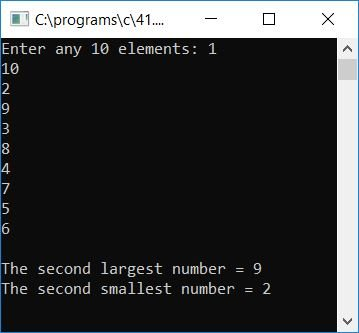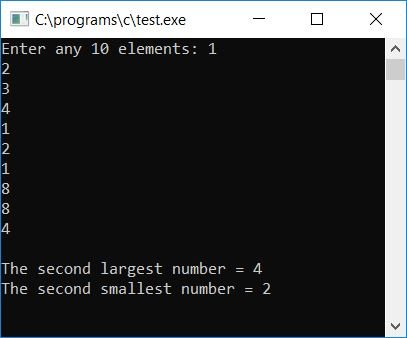# C Program to Print the Second Largest and Second Smallest Array Element

In this tutorial, we will learn how to create a program in C that will ask the user to enter 10 array elements as input and then find and print out the second largest and second smallest element from that given array. Here is the program:

```#include<stdio.h>
#include<conio.h>
int main()
{
int arr, great, small, i, j, k, limit=10, count=0;
printf("Enter any 10 elements: ");
for(i=0; i<limit; i++)
{
scanf("%d", &arr[i]);
}

// remove the duplicate element
for(i=0; i<limit; i++)
{
for(j=i+1; j<limit; j++)
{
if(arr[i]==arr[j])
{
count++;
limit = limit-count;
for(k=j; k<limit; k++)
arr[k] = arr[k+1];
count = 0;
}
}
}

// find the first largest and smallest element
great = arr;
small = arr;
for(i=0; i<limit; i++)
{
if(great<arr[i])
great = arr[i];
if(small>arr[i])
small = arr[i];
}

// remove the first largest element
for(i=0; i<limit; i++)
{
if(great==arr[i])
{
count++;
limit = limit-count;
for(j=i; j<limit; j++)
arr[j] = arr[j+1];
count = 0;
}
}

// remove the first smallest element
for(i=0; i<limit; i++)
{
if(small==arr[i])
{
count++;
limit = limit-count;
for(j=i; j<limit; j++)
arr[j] = arr[j+1];
count = 0;
}
}

// now find the largest and smallest element, this time it will be the second largest/smallest
great = arr;
small = arr;
for(i=0; i<limit; i++)
{
if(great<arr[i])
great = arr[i];
if(small>arr[i])
small = arr[i];
}
printf("\nThe second largest number = %d", great);
printf("\nThe second smallest number = %d", small);
getch();
return 0;
}```

The program was built and run in the Code::Blocks IDE. Here is the first snapshot of the sample run:Provide any 10 elements and then press the ENTER key to see the output that will print the second largest and the second smallest element from the list of given 10 numbers as array elements, as shown in the second snapshot of the sample run given here:Here is another sample run of the above program. Assume the user has provided some duplicate elements as input this time, say 1, 2, 3, 4, 1, 2, 1, 8, 8, and 4 are the 10 numbers provided by the user at run-time. As you can see, there are some duplicate elements in the following way:

• 1 present three times
• 2 present two times
• 3 present one time
• 4 present two times
• 8 present two times

Therefore, the above program will first remove all the duplicate elements and then find out the first largest and smallest element, and then remove both to find the largest and smallest element that will be the second largest and smallest element of the given element. In this case, the second largest element will be 4, and the second smallest element will be 2. This is the final snapshot of the sample run:Here are some of the main steps used in the above program:

• Receive any 10 numbers as 10 array elements.
• Remove all the duplicate elements from the array.
• To remove duplicate elements from the array, follow these steps:
• Take a variable, say limit, at the start of the program and initialize it with 10 (this is the size of the array).
• Create a for loop that runs from 0 to one less than the value of the limit variable.
• Inside this for loop, create another for loop that starts from one greater than the value of i (the outer loop variable), that is i+1, and runs up to one less than the value of variable limit.
• Compare the element present at the ith index with the element present at the jth index, that is, whether they are equal or not.
• If they are equal to each other, then remove that element and continue to check for the next match.
• Now find the first largest and smallest element.
• Here are the steps to finding the first largest and smallest element:
• Set the first element to be the largest and smallest.
• Now compare the largest element to every other element in the array to see if it is less than any other element.
• If it is found to be lesser, then place that element or initialize that element as the largest element and continue to check for the next.
• Repeat the process to find the first smallest element.
• Now remove the first largest and smallest element from the given array.
• Find the largest and smallest elements in the given array once more.
• As the first largest and smallest element were deleted, this time the largest and smallest element will be the second largest and smallest element.
• The user can print the value of both the variables "great" and "small" that hold the second largest and second smallest element from the given array at run-time.

C Quiz

« Previous Program Next Program »